# Market Power: Monopoly and Monopsony

Boost your grades with best homework help from our qualified and skilled subject experts## Why Students Order Homework Help From Us### Last Minute Request

Our experts solve complex problems with high accuracy, ensuring fast, reliable help without compromise.### Custom Essay Writing

Professional essays for all subjects and degrees, guaranteed for accuracy, authenticity, and originality.### AI & Plagiarism-Free

Our skilled writers turn your ideas into 100% original, plagiarism- & AI-free papers.### Transparent Pricing

Market Power: Monopoly and Monopsony

A market in which buyers and sellers have all the information about product and it makes comparison of prices easy as they are identical; we call it as Perfectly Competitive Market. There are many buyers and many sellers in this market. There are no barriers to entry or exit in such market.

Monopoly and Monopsony are equally opposite of Perfect competition. In this context, a Monopoly is a kind of market which has one seller but several buyers. A Monopsony can be said just opposite of monopoly, as in this kind of market there is many sellers but only one buyer. But Monopoly and Monopsony are related to each other.

Let us deliberate the behavior of a Monopolist. A monopolist can be said a price maker. He decides the price of the good to be sold. He can change the price or quantity of the goods. It can be higher quantity at a low price in a very elastic market and lower quantity at a high price in a less elastic market. Another characteristic of monopolies is there is no need of advertising product to increase share in market. They use the channel of public relation and maintain good relationship with their buyers. There are no close substitutes of the product in monopoly kind of market. For example, providers of water, natural gas, telecommunications and electricity are granted rights to provide service through local government is a perfect example of monopoly. A monopolist should shut down when it becomes impossible to cover the variable cost. In such case, the demand curve is wholly below the average variable cost curve. Under these situations, average revenue would be less than average variable costs and this suggests the monopolist to shut down.

Pure monopoly is infrequent.In many markets, competition is only between few firms. Like there is a company which is a single source of a product with no close substitutes. The structure of such market is complex and difficult to interact among the firms. Strategies play a role in such monopoly and firms can charge higher price as they find it good moneymaking. To maintain pure monopoly, there must be barriers like legal barriers, control of resources, economies of scale, etc., for the competitors to enter the market.

The next type of market is to discuss is Monopsony. It is a type of market structure in which there are many sellers and one buyer interacts with them. Here, single buyer controls the market. Just like monopolist, a monopsony employer finds it profitable if prices are discriminated. It simply means paying different wages to different group of workers even if Maximum Retail Price is same. Monopsonies take different size and shapes, but commonly it takes place when a single employer controls the entire labor market. Technologies can be considered as a perfect example of Monopsony.

By considering how the choices are being made, we can validate the close relation between monopoly and monopsony. Pure monopsony is rare, a buyer’s monopsony is a situation in which buyer can ask for concessions from the seller. Sellers have no choice other than selling products to the buyer. These buyers have monopsony powers. We will discuss about monopsony power, also its measurement, and price effects.

10.1 Monopoly

Monopolists do not think about their competitors, they hold an exceptional position. They don’t worry about who are decreasing their prices to sell products. If a monopolist wants to increase the price of the product, he can do so without thinking about the market’s share of the competitors. Being a sole producer a monopolist can control the output presented for sale.

Characteristics of a Monopolist-

• Monopolists can be called profit maximizer as they focus on maximizing profit.
• Monopolists decides the price of the goods which is to be sold by them, we can say they are price maker.
• Other sellers cannot enter the market of monopoly.
• They can be said as a single seller.
• A monopolist can modify the price or quantity of the product.

Entering the market of monopoly is restricted. There are three major barriers- Economic, deliberate and legal barriers. Addition to these barriers there is exit barrier. Barriers to exit means the condition of market in which it is difficult for the company to end its existence in market or to quit from market. A company will shut down if price decreases below minimum average variable costs.

Controlling the price by monopolists does not mean that they can charge any value for price to maximize profit. For this, the cost and characteristics must be examined or determined. To make a decision regarding price of products, knowledge of costs and demand must be known. The monopolist analyze the profit output maximized by profit through finding that quantity where marginal revenue equals marginal costs, then notice the market demand curve quantity to decide what market price resembles to that quantity.

Shifts in Demand

There is a rich bond between price and quantity supplied in competitive market. This is defined by supply curve. How much to produce at given price is what supply curve shows. There is no supply curve in monopoly because the decision of output depends on marginal cost and shape of demand curve.

Thus we can say that shifts in demand do not show the prices and quantities which agrees to supply curve. We can be clear in this by referring the following figure-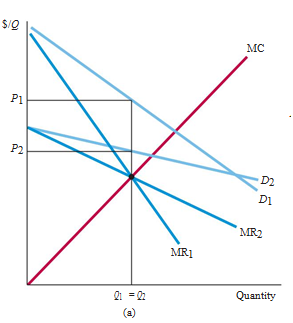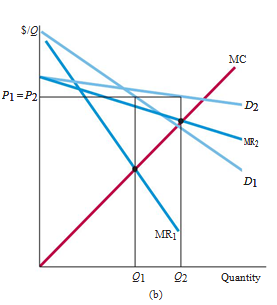The upward movement of demand shows the shifting of market with new equilibrium. This will be in short run and might offer higher price along with the possibility of lower quantity. In monopoly market, the new market equilibrium will shift the market towards larger quantity of goods produced so that it can remain in good condition. We can see the example by looking at the above figures and both highlights the principle of demand curve. We can even see that the shifting of demand curve offers the new marginal curve and revenue curve of the firm.

In the above figure, the line MR2 gets intersected with marginal cost curve MR1 line. This is the actual reason of shifted results in the firm. Price might fall or rise and with this, the curve plotted above shifts.

In case, if these two curves are overlapped with each other and such that the larger quantity will be found, price might not be charged more. But the shift changes the values of price and quantity at the same time. For special cases, the above plotted graph shoes the difference between monopoly as well as competitive market. If the firm supplies several quantities of goods, in that case, the monopolist power will shift the market.

A Rule Of Thumb for Pricing

As we know price and output must be decided keeping in mind marginal revenue should be equal to marginal cost, it is important to know that how manager of the firm could find correct price and output level? Restricted knowledge of average curve and marginal revenue curve by the managers create difficulties.

Mathematically, the relationship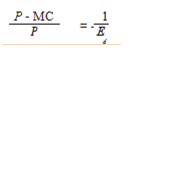It is the rule of thumb for pricing where (P – MC)/P indicates markup over marginal cost and negative Ed shows inverse of elasticity of demand.

Note:

It means if demand is elastic, this will be beneficial for monopolist. Remember that it is not possible for monopolist to produce output on the inelastic region of demand curve (the area where the value of demand is less than one in the absolute value).

Suppose monopolist produce output at a point in the demand curve – 0.5, it is possible to acquire greater amount of profit. For this, theproduction should be less and selling of the item should be at higher cost price.Here, the shifting of demand curve towards the value more than one in the absolute value is possible. This means it satisfies markup rule of equation.

If we consider the marginal cost becomes zero (anyhow), the equation cannot be used directly for obtaining profit-maximizing price.Here, we should take the point at which elasticity of demand is equal to –1 to produce the output. So, in this case, the value of maximizing profit will be equal to maximizing revenue. It means the revenue gets maximized if the value of Ed = –1.

The Effect of Tax

Generally the amount of tax is less than the rise in market price per unit in competitive industry. Considering the fact, if burden of tax is shared by consumers and the producers, the effect of tax is different in case of monopoly. Under Monopoly, price rises more than the value of tax amount sometimes. Let us analyze the effect of tax in case of monopoly. It is simple to understand the effect. Let’s take tax as t dollars per unit, which monopolists pay to the government according to the units he sold. By this the firm’s marginal and average cost will increase by the tax amount i.e., t. if MC is the firm’s original marginal cost then its production decision can be explained as-

MR = MC + t

The effect of tax on a monopolist can be explained by the following figure-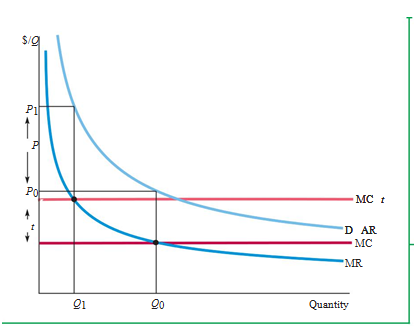We can see the marginal cost curve is shifted by an amount of tax t, and new intersection formed. The figure shows Q0 and P0 as quantity and price respectively before the tax is levied, and Q1 and P1 are the quantity and price after the tax. The upward shifting of marginal cost curve gives a result of rise in price with smaller quantity. Sometimes price rise is less than the amount of tax, but in figure we can see price increases more than the tax amount. It can only happen in case of monopoly. It is not possible in competitive market as the connection between price and marginal cost rely on the demand elasticity.

The Multiplant Firm

As we know a firm makes profit by backgroundproductionwherever marginal revenue equals to marginal cost. The term Multiplant firm means production process takes place in more than one place whose operational costs can be differ from each other. Choosing production levels is same as it is in one plant firm. Consider a firm has more than one plant, say two plants. In this case, what should be the total output of the firm and how much of this output value should each of these plants yield? We can get the answer by these two steps-

Step 1- The total output will then divided so that the value of marginal cost remains same in each of two plants. If we don’t divide the total output then the firm could decrease its prices and raising its profit by transferring production. Let’s take an example to clear the view; if marginal cost of 1st plant is higher than the 2nd, the firm could generate the similar level of results that too at a lower value just by producing less at 1st and more at 2nd plant.

Step 2- As we know, the total output should be at a level, the marginal revenue will be equal to marginal cost. For example, consider that marginal costs are same, but marginal revenue beaten marginal cost at each plant. In such situation, the firm would prefer manufacturing more at both the plants since the revenue made from additional units and this would outstrip the price. As marginal costs kept same, and marginal revenue will be equal to marginal cost, we notice that profit gets maximized when the marginal revenue becomes equal to marginal cost.

10.2 Monopoly Power

Pure monopoly can be said as a single supplier. Monopoly power is much widespread in such case. Monopolies are formed under following conditions-

• When a firm has special ownership such as telecom businesses.
• When monopoly permission granted by government such as post office.
• When firms are in possession of copyrights and patents providing them special rights to sell a product such as Microsoft windows.
• When firms merge to hold a position in market.

The monopoly power can be maintained by maintain barriers to exit which includes, economies of large scale production, dropping of price too low in a demonstration of power and put pressure on the competitors, limit pricing, by ownership of scarce resources, high set up cost, high expenditure on advertising, maintaining brand loyalty, and by taking exclusive contracts to restrict the entry of other retailers.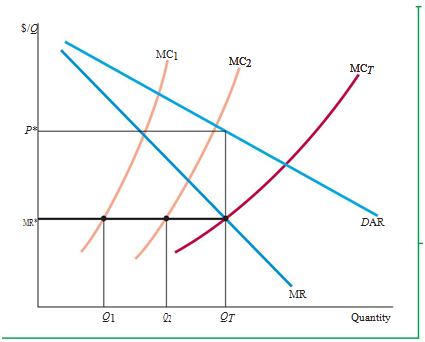For example, there are four firms that are producing toothbrushes. If these four firms produce a total of 20,000 units on an aggregate daily which gives the result to 5000, if they set the price of toothpaste to \$1.50 then its demand curve will be obtained as inelastic. In this case, the factor will give the elasticity of demand as a decision to get the value of –1.5.

In case first firm is willing to lower the price and increase its sales, then we can see changes in the demand curve which leads to oppose the previous value of its market demand curve.

When any of the firm decides to increase the price of toothpaste to reach \$1.60, the demand curve will again shift and the numbers of consumers will be changed. It is likely that if the quality of the product does not change than its sales will be affected. Whatsoever the condition is, it is true that sales will never come to zero. Considering the competitors, the number of consumers they have varied from this firm. And there is a possibility that any other will also raise the prices to make the things a bit change.

Taking the first case, if the firm has lowered the price by \$0, it will set to \$1.40. This is obvious that it increases the sale as well as market revenue without affecting it. But, there is no certainty whether this firm would capture the whole market or not. In this situation, the demand curve will depend on market demand and the existence of products of other firms. If the quality of the toothpaste is dissimilar, then the best with quality will lead the market. This tells the story of entirely competitive market.

Production, Price and Monopoly Power

Determination of elasticity of demand and market demand is not difficult when the firm’s product is in the market shows better output. They do research and find out the variation in demand so that their demand curve can be shifted to offer more profit.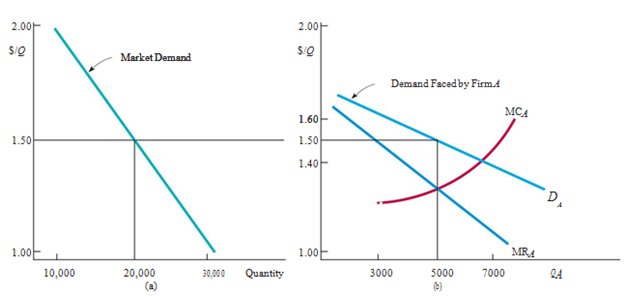Considering the figure (a) in which the Firm A knows how to shift its market as per the conditions and know the details of market demand curve. But the fact is, how Firm A will do production to earn profit? In this context, profit-maximization is a big factor which is related to marginal revenue as well as marginal cost of the firm.

In figure (b), we can see that 5000 units are produced and the prices are for \$1.50. It indicates that this price has already exceeds marginal costs.The presence of monopoly power will be seen when this price becomes higher than the value of marginal cost. Suppose the power is quite less and have no potential to drive the market so the firm might drive away. Here two different questions arise that include- how monopoly power should be compared and why firms should have high monopoly power than its competitors? The answer is- to make a balance in the market so that they can stand there.

Measuring Monopoly Power

There is an important dissimilarity between competitive firm and those firms with monopoly power. For the competitive firm the price equals to the marginal cost. In case of monopoly power of any firm, the price will be exceeded to marginal cost. Thus, most of the time, firms changes their strategy and apply the law of thumb for valuing. This is how the existence of these firms is there and holds better revenue.

The transformation between price & marginal cost is divided by price. This value can also be represented mathematically to show its present scenario. Taking the help of Lerner Index, the value will always show below one and above zero. In case, the value of Lerner Index is large, then it is possible to have higher degree of monopoly control. When these facts are calculated and plotted in graphs, it can also be considered as the elasticity of demand. Remember, in this case the value will be for elasticity of demand for firm. So the elasticity of market demand is not considered.

We have previously seen the example of toothpaste. If we consider the elasticity of demand and degree of monopoly power, the later might not produce any higher amount of profits. But average cost with respect to the price changes can offer this higher value of profit. Comparing Firm A and Firm B, the higher value of monopoly with Firm A. In this case, Firm B will earn lower amount of profit because of the higher average costs. And the amount of profit to Firm A will remain greater than Firm B still it is in monopoly power.

The Rule Of Thumb for Pricing

For determining the value of price changes to markup over marginal cost, the equation will be given by-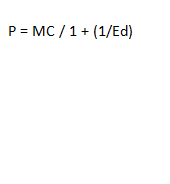The above relationship helps in calculating the value of rule of thumb when the firm has monopoly power. In this case, Ed refers elasticity of demand. No one should confuse about elasticity of market demand which was previously used. It reflects the story how the competitors of the market will act so that the unit sale can be increased at every point of time by just making 1% change in price. Every firm follows similar kind of strategies.